# Chemistry Notes for Class 11 STRUCTURE OF ATOM Download in pdf

Chapter 2 Structure Of Atom

#### Aoms:

Atom is the smallest indivisible particle of the matter. Atom is made of electron, proton and neutrons.

 PARTICLE ELECTRON PROTON NEUTRON Discovery Sir. J. J. Thomson (1869) Goldstein (1886) Chadwick (1932) Nature of charge Negative Positive Neutral Amount of charge 1.6 x 10-19Coloumb 1.6 x 10-19Coloumb 0 Mass 9.11 x 10-31kg 1.672614 x 10-27kg 1.67492 x10-27kg

Electrons were discovered using cathode ray discharge tube experiment.

Nucleus was discovered by Rutherford in 1911.

Cathode ray discharge tube experiment: A cathode ray discharge tube madeof glass is taken with two electrodes. At very low pressure and high voltage,current starts flowing through a stream of particles moving in the tube fromcathode to anode. These rays were called cathode rays. When a perforatedanode was taken, the cathode rays struck the other end of the glass tube atthe fluorescent coating and a bright spot on the coating was developed

#### Results of Rutherford experiments:

a. Cathode rays consist of negatively charged electrons.
b. Cathode rays themselves are not visible but their behavior can be observed with help of fluorescent or phosphorescent materials.
c. In absence of electrical or magnetic field cathode rays travel in straight linesd. In presence of electrical or magnetic field, behaviour of cathode rays is similar to  that shown by electrons
e. The characteristics of the cathode rays do not depend upon the material of the electrodes and the nature of the gas present in the cathode ray tube.

Charge to mass ratio of an electron was determined by Thomson. The chargeto mass ratio of an electron as 1.758820 x 1011. C kg-1

Charge on an electron was determined by R A Millikan by using an oil dropexperiment. The value of the charge on an electron is -1.6 x 10-19C.

The mass on an electron was determined by combining the results ofThomson’s experiment and Millikan’s oil drop experiment. The mass of anelectron was determined to be 9.1094 x 10-31kg.

###### Discovery of protons and canal rays:

Modified cathode ray tube experimentwas carried out which led to the discovery of protons.

###### Characteristics of positively charged particles:

a. Charge to mass ratio of particles depends on gas from which these originateb. The positively charged particles depend upon the nature of gas present in the cathode ray discharge tube
c. Some of the positively charged particles carry a multiple of fundamental of electrical charge.d. Behaviour of positively charged particles in electrical or magnetic field is opposite to that observed for cathode rays

Neutrons were discovered by James Chadwick by bombarding a thin sheet of beryllium by α- particles. They are electrically neutral particles having a mass slightly greater than that of the protons.

Atomic number (Z) : the number of protons present in the nucleus(Moseley1913).

Mass Number (A) :Sum of the number of protons and neutrons present in thenucleus.

##### Thomson model of an atom:

This model proposed that atom is considered asa uniform positively charged sphere and electrons are embedded in it.An important feature of Thomson model of an atom was that mass of atom isconsidered to be evenly spread over the atom.Thomson model of atom is also called as Plum pudding, raisin pudding orwatermelon model Thomson model of atom was iscarded because it could not explain certainexperimental results like the scattering of α- particles by thin metal foils.

#### Observations from α- particles scattering experiment by Rutherford:

a. Most of the α- particles passed through gold foil un deflected
b. A small fraction of α- particles got deflected through small angles
c. Very few α- particles did not pass through foil but suffered large deflection nearly180o

#### Conclusions Rutherford drew from α- particles scattering experiment:

a.  Since most of the α-particles passed through foil undeflected, it means most of the  space in atom is empty
b.  Since some of the α-particles are deflected to certain angles, it means that there is positively mass present in atom
c.  Since only some of the α-particles suffered large deflections, the positively charged mass must be occupying very small space
d.  Strong deflections or even bouncing back of α-particles from metal foil were due to  direct collision with positively charged mass in atom

## Rutherford’s model of atom :

This model explained that atom consists ofnucleus which is concentrated in a very small volume. The nucleus comprisesof protons and neutrons. The electrons revolve around the nucleus in fixedorbits. Electrons and nucleus are held together by electrostatic forces ofattraction.

### Drawbacks of Rutherford’s model of atom :

a.  According to Rutherford’s model of atom, electrons which are negatively
charged particles revolve around the nucleus in fixed orbits. Thus,
b.  theelectrons undergo acceleration. According to electromagnetic theory of Maxwell, a charged particle undergoing acceleration should emitelectromagnetic radiation. Thus, an electron in an orbit should emitradiation. Thus, the orbit should shrink. But this does not happen.c.  The model does not give any information about how electrons aredistributed around nucleus and what are energies of these electrons

Isotopes: These are the atoms of the same element having the same atomicnumber but different mass number.e g 1H1,1H2,1H3

Isobars: Isobars are the atoms of different elements having the same massnumber but different atomic number.e g 18Ar40 , 20Ca40

Isoelectronic species: These are those species which have the same numberof electrons.

The radiations which are associated withelectrical and magnetic fields are called electromagnetic radiations. When anelectrically charged particle moves under acceleration, alternating electricaland magnetic fields are produced and transmitted. These fields aretransmitted in the form of waves. These waves are called electromagneticwaves or electromagnetic radiations.

a.  Oscillating electric and magnetic field are produced by oscillating charged particles. These fields are perpendicular to each other and both areperpendicular to the direction of propagation of the wave.
b.  They do not need a medium to travel. That means they can even travel invacuum.

a.  Wavelength: It may be defined as the distance between two neighbouring crests or troughs of wave as shown. It is denoted by λ.

b.  Frequency (ν): It may be defined as the number of waves which passthrough a particular point in one second.

c.  Velocity (v): It is defined as the distance travelled by a wave in onesecond. In vacuum all types of electromagnetic radiations travel with thesame velocity. Its value is 3 ×10 8m sec-1. It is denoted by v
d. Wave number: Wave number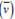is defined as the number of wavelengths per unit length.
Velocity = frequency ×wavelength    c = νλ

### Planck's Quantum Theory-

o The radiant energy is emitted or absorbed not continuously but discontinuously in the form of small discrete packets of energy called ‘quantum’. In case of light , the quantum of energy is called a ‘photon’
o The energy of each quantum is directly proportional to the frequency of the radiation, i.e. E α υ     or E= hυ      where h= Planck’s constant = 6.626 x 10-27 Js
o Energy is always emitted or absorbed as integral multiple of this uantum. E=nhυ Where n=1,2,3,4,.....

Black body: An ideal body, which emits and absorbs all frequencies, is calleda black body. The radiation emitted by such a body is called black body radiation.

### Photoelectric effect:

The phenomenon of ejection of electrons from thesurface of metal when light of suitable frequency strikes it is calledphotoelectric effect. The ejected electrons are called photoelectrons.

#### Experimental results observed for the experiment of Photoelectric effect-

o When beam of light falls on a metal surface electrons are ejectedimmediately.
o Number of electrons ejected is proportional to intensity or brightness of light
o Threshold frequency (vo): For each metal there is a characteristicminimum frequency below which photoelectric effect is not observed. Thisis called threshold frequency.
o If frequency of light is less than the threshold frequency there is noejection of electrons no matter how long it falls on surface or how high isits intensity.

Photoelectric work function (Wo): The minimum energy required to ejectelectrons is called photoelectric work function.Wo= hvo

Energy of the ejected electrons :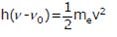#### Dual behavior of electromagnetic radiation

The light possesses both particle and wave like properties, i.e., light has dual behavior . whenever radiation interacts with matter, it displays particle like properties.(Black body radiation and photoelectric effect) Wave like properties are exhibited when it propagates(interference an diffraction)

### Spectrm :-

When a white light is passed through a prism, it splits into a series ofcoloured bands known as spectrum.

Spectrum is of two types:

(a) Continuous and line spectrum  The spectrum which consists of all the wavelengths is called continuous
spectrum.
(b) line spectrum A spectrum in which only specific wavelengths are present is known as a line spectrum. It has bright lines with dark spaces between them.

Electromagnetic spectrum is a continuous spectrum. It consists of a range ofelectromagnetic radiations arranged in the order of increasing wavelengths ordecreasing frequencies. It extends from radio waves to gamma rays.

Spectrum is also classified as emission and line spectrum.

Emission spectrum: The spectrum of radiationemitted by a substance that has absorbed energy is called an emissionspectrum.
o Absorption spectrum is the spectrum obtained when radiation is passedthrough a sample of material. The sample absorbs radiation of  certainwavelengths. The wavelengths which are absorbed are missing and comeas dark lines.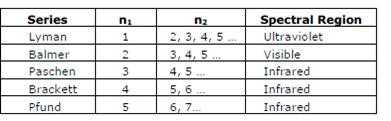The study of emission or absorption spectra is referred as spectroscopy.

Spectral Lines for atomic hydrogen:

Rydberg equation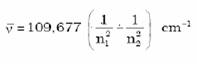R = Rydberg’s constant = 109677 cm-1

### Bohr’s model for hydrogen atom:

a. An electron in the hydrogen atom can move around the nucleus in a circular path of fixed radius and energy. These paths are called orbits orenergy levels. These orbits are arranged concentrically around thenucleus
b.  As long as an electron remains in a particular orbit, it does not lose or gain energy and its energy remains constant.
c.  When transition occurs between two stationary states that differ inenergy, the frequency of the radiation absorbed or emitted can be calculated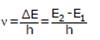d.  An electron can move only in those orbits for which its angularmomentum is an integral multiple of h/2π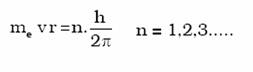The radius of the nth orbit is given byrn =52.9 pm xn2/Z
energy of electron in nth orbit is :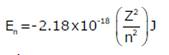Limitations of Bohr’s model of atom:

a.  Bohr’s model failed to account for the finer details of the hydrogen spectrum.
b.  Bohr’s model was also unable to explain spectrum of atoms containing more than one electron.

#### Dual behavior of matter:

de Broglie proposed that matter exhibits dualbehavior i.e. matter shows both particle and wave nature. de Broglie’s relation is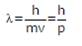#### Heisenberg’s uncertainty principle:

It states that it is impossible to determine simultaneously, the exact position and exact momentum (or velocity) of an electron.The product of their uncertainties is always equal to or greater than h/4π.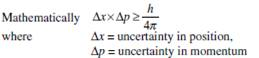Heisenberg’s uncertainty principle rules out the existence of definite pathsor trajectories of electrons and other similar particles

#### Failure of Bohr’s model:

a. It ignores the dual behavior of matter.

b. It contradicts Heisenberg’s uncertainty principle.

Classical mechanics : is based on Newton’s laws of motion. It successfullydescribes the motion of macroscopic particles but fails in the case ofmicroscopic particles.Reason: Classical mechanics ignores the concept of dual behaviour of matter especially for sub-atomic particles and the Heisenberg’s uncertainty principle.

Quantum mechanics is a theoretical science that deals with the study of themotions of the microscopic objects that have both observable wave like andparticle like properties.
Quantum mechanics is based on a fundamental equation which is calledSchrodinger equation.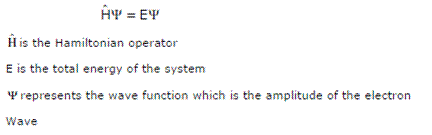#### Schrodinger’s equation:

For a system (such as an atom or a molecule whoseenergy does not change with time) the Schrödinger equation is written as:

When Schrödinger equation is solved for hydrogen atom, the solution givesthe possible energy levels the electron can occupy and the correspondingwave function(s) of the electron associated with each energy level.Out of the
possible values, only certain solutions are permitted. Eachpermitted solution is highly significant as it corresponds to a definite energystate. Thus, we can say that energy is quantized.

Probability density :  ψ gives us the amplitude of wave. The value of ψhas no physicalsignificance. Ψ2gives us the region in which the probability of finding an electron ismaximum. It is called probability density.

Orbital: The region of space around the nucleus where the probability offinding an electron is maximum is called an orbital.

#### Quantum numbers:

There are a set of four quantum numbers which specifythe energy, size, shape and orientation of an orbital. To specify an orbital only three quantum numbers are required whileto specify an electron all four quantum numbers are required.

Principal quantum number (n):It identifies shell, determines sizes and energy of orbitals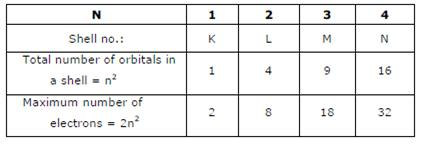Azimuthal quantum number (l): Azimuthal quantum number. ‘l’ is also known as orbital angular momentum or subsidiary quantum number. l. It identifies sub-shell, determines the shape of orbitals, energy of orbitals in multi-electron atoms along with rincipal quantum number and orbital angular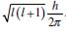momentum, i.e.,  The number of orbitals in a subshell = 2l + 1. For a given value of n, it can have n values ranging from 0 to n-1. Total number of subshells in a particular shell is equal to the value of n.

 Subshell notation s p d f g Value of ‘l’ 0 1 2 3 4 Number of orbitals 1 3 5 7 9

Magnetic quantum number or Magnetic orbital quantum number (ml): Itgives information about the spatial orientation of the orbital with respect tostandard set of co-ordinate axis.For any sub-shell (defined by ‘l’ value) 2l+1 values of ml are possible.For each value of l, ml = – l, – (l –1), – (l–2)... 0,1...(l – 2), (l–1), l

Electron spin quantum number (ms):It refers to orientation of the spin of theelectron. It can have two values +1/2 and -1/2. +1/2 identifies the clockwisespin and -1/2 identifies the anti- clockwise spin.

#### Nodal surfaces or simply nodes :

The region where this probability density function reduces to zero is called nodal surfaces or simply nodes.

Radial nodes: Radial nodes occur when the probability density of wave functionfor the electron is zero on a spherical surface of a particular radius. Numberof radial nodes = n – l – 1

Angular nodes: Angular nodes occur when the probability density wavefunction for the electron is zero along the directions specified by a particularangle. Number of angular nodes = l
Total number of nodes = n – 1

Degenerate orbitals: Orbitals having the same energy are called degenerateorbitals. Shape of p and d-orbitals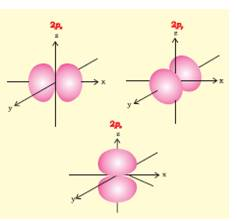#### Shielding effect or screening effect:

Due to the presence of electrons in theinner shells, the electron in the outer shell will not experience the full positivecharge on the nucleus. So, due to the screening effect, the net positive charge experienced by theelectron from the nucleus is lowered and is known as effective nuclear charge. Effective nuclear charge experienced by the orbital decreases with increase of azimuthal quantum number (l).

##### Aufbau Principle:

In the ground state of the atoms, the orbitals are filled inorder of their increasing energies

n+l rule-Orbitals with lower value of (n+l) have lower energy. If two orbitals have the same value of (n+l) then orbital with lower value of nwill have lower energy.
The order in which the orbitals are filled isas follows:
1s, 2s, 2p, 3s, 3p, 4s, 3d, 4p, 5s, 4d, 5p, 4f, 5d, 6p, 7s...

##### Pauli Exclusion Principle:

No two electrons in an atom can have the same setof four quantum numbers. Only two electrons may exist in the same orbitaland these electrons must have opposite spin.

##### Hund’s rule of maximum multiplicity:

Pairing of electrons in the orbitalsbelonging to the same subshell (p, d or f) does not take place until eachorbital belonging to that subshell has got one electron each i.e., it is singlyoccupied.

##### Electronic configuration of atoms:

Arrangement of electrons in different orbitals of an atom. The electronic configuration of differentatoms can be represented in two ways. a. sapbdc...... notation.

b. Orbital diagram:, each orbital of the subshell is represented by a box and the electron is  represented by an arrow (↑) a positive spin or an arrow (↓) a negative spin.

#### Stability of completely filled and half filled subshells:

a.  Symmetrical distribution of electrons- the completely filled or half filled sub-shells have symmetrical distribution of electrons in them and are more stable.

b.  Exchange energy-The two or more electrons with the same spin present in the degenerate orbitals of a sub-shell can exchange their position and the energy released due to this exchange is called exchange energy. The number of exchanges is maximum when the subshell is either half filled or completely filled. As a result the exchange energy is maximum and so is the stability.

### NCERT Books Free Pdf Download for Class 5, 6, 7, 8, 9, 10 , 11, 12 Hindi and English Medium

 Mathematics Biology Psychology Chemistry English Economics Sociology Hindi Business Studies Geography Science Political Science Statistics Physics Accountancy